# The power of origami

December 2009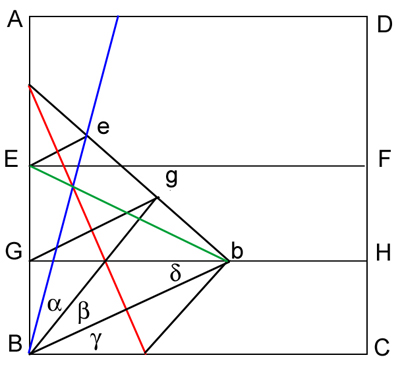### Trisecting the angle using origami — proof

In the image on the right the angle between the blue line and the bottom edge is the angle to be trisected, and we must show that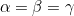.

The red line is the crease resulting from the fold in step 4 of the folding sequence. Now look at the triangle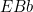. We know that the length of the line segment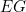is equal to the length of the line segment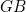, and we also know that the line segment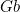, which is the height of the triangle, meets the line segment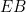at a right angle. In other words, the heightof the triangle divides the opposite side into half. This means that the triangleis isosceles.

The mirror image of the trianglewhen reflected in the red crease line is the triangle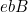, which is therefore also isosceles.

The height of the triangleextending from the point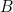therefore divides the angle atinto half. This shows that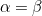. By mirror symmetry we have that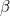is equal to the angle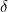of the triangle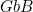. And since the line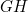is parallel to the bottom edge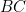, we have that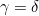. This proves that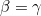.